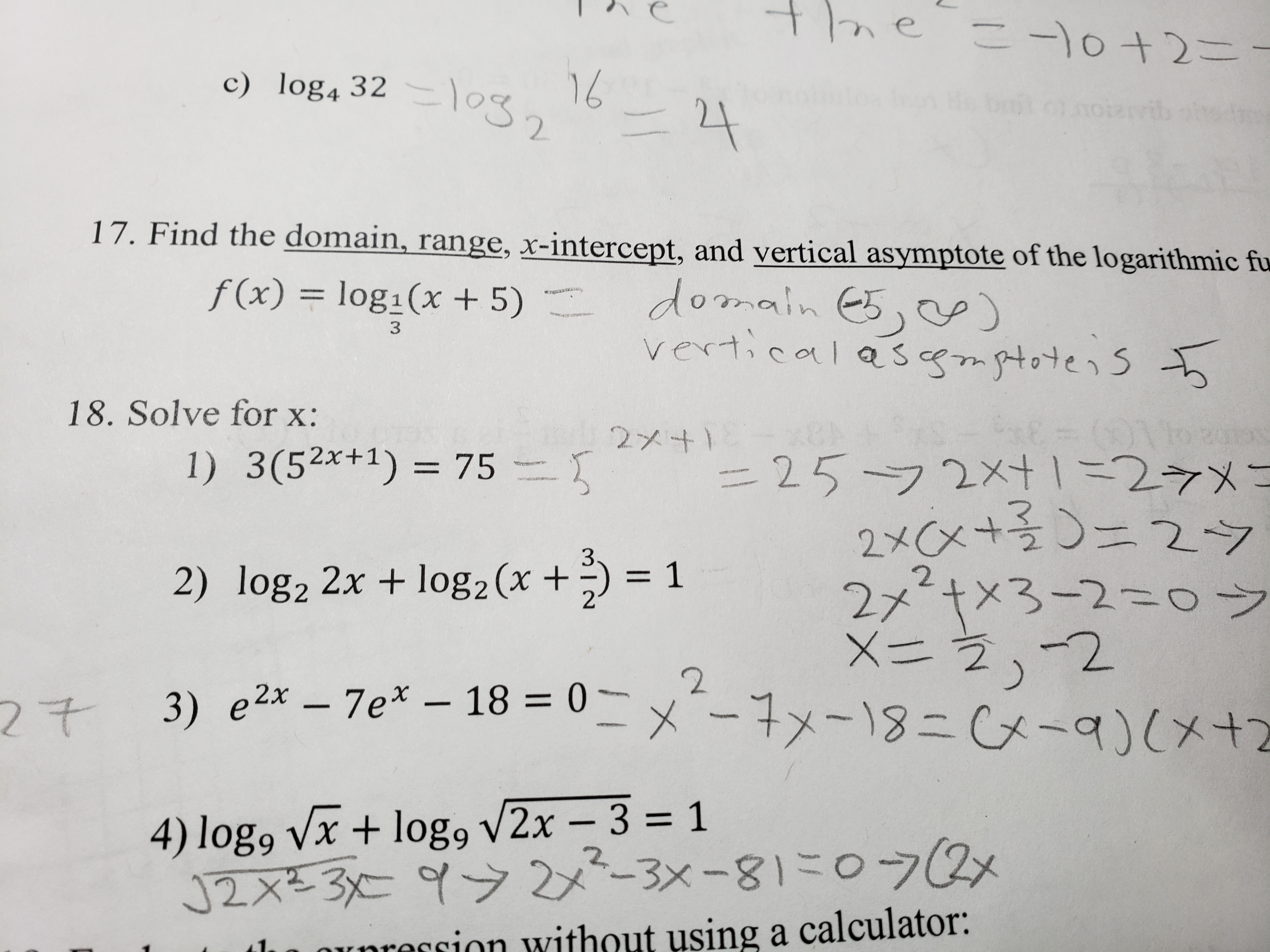# c) log4 3217. Find the domain, range, x-intercept, and vertical asymptote of the logarithmic fuf(x) = log-(x + 5) ·domain es,。。)18. Solve for x:2x41) = 752) 1082 2x + 1082 (x + 3) = 12x21x3-2二 フ4) log, v/X + log9V 2x-3 = 1unrersion without using a calculator:

Question

4help_outlineImage Transcriptionclosec) log4 32 17. Find the domain, range, x-intercept, and vertical asymptote of the logarithmic fu f(x) = log-(x + 5) · domain es,。。) 18. Solve for x: 2x41) = 75 2) 1082 2x + 1082 (x + 3) = 1 2x21x3-2 二 フ 4) log, v/X + log9V 2x-3 = 1 unrersion without using a calculator: fullscreen
check_circleExpert Solution
Step 1
Step 2

Solve the given equation and find the value of x.

Step 3

Use the quadratic equation formula to solve the...

### Want to see the full answer?

See Solution

#### Want to see this answer and more?

Solutions are written by subject experts who are available 24/7. Questions are typically answered within 1 hour*

See Solution
*Response times may vary by subject and question
Tagged in

### Other